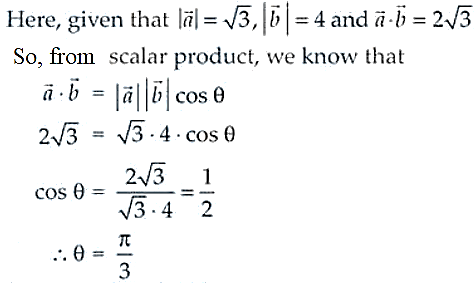# NCERT Exemplar Solutions for Class 12 Maths Chapter 10 Vector Algebra

The NCERT Exemplar textbooks are important for students in order to attain strong conceptual knowledge. They can now access the NCERT Exemplar Solutions, available subject-wise, for guidance to solve the exemplar problems. All the solutions are crafted to help students prepare well for the board exam. Further, all solutions are created by subject matter experts, following the latest CBSE syllabus.

The 10th Chapter of NCERT Exemplar Solutions for Class 12 Mathematics is Vector Algebra. Here, students will learn the introduction to vectors, their types, addition and multiplication performed on vectors, components of the vector, section formula, scalar product, projection of a vector on a line and vector product. To attain a strong grip over the concepts of this chapter, students can make use of the solutions PDF of NCERT Exemplar Solutions for Class 12 Maths Chapter 10 Vector Algebra from the link given below.

## Download the PDF of NCERT Exemplar Solutions for Class 12 Maths Chapter 10 Vector Algebra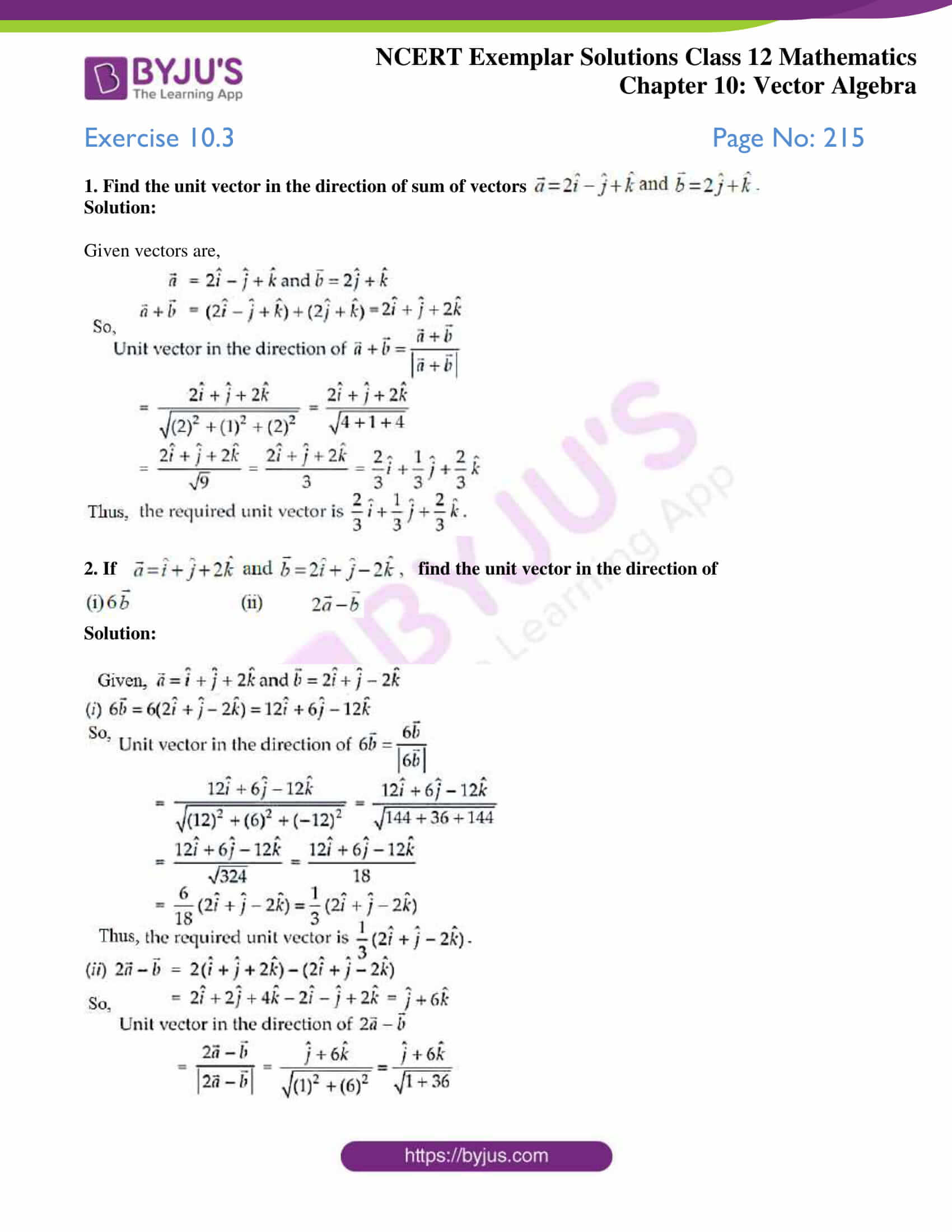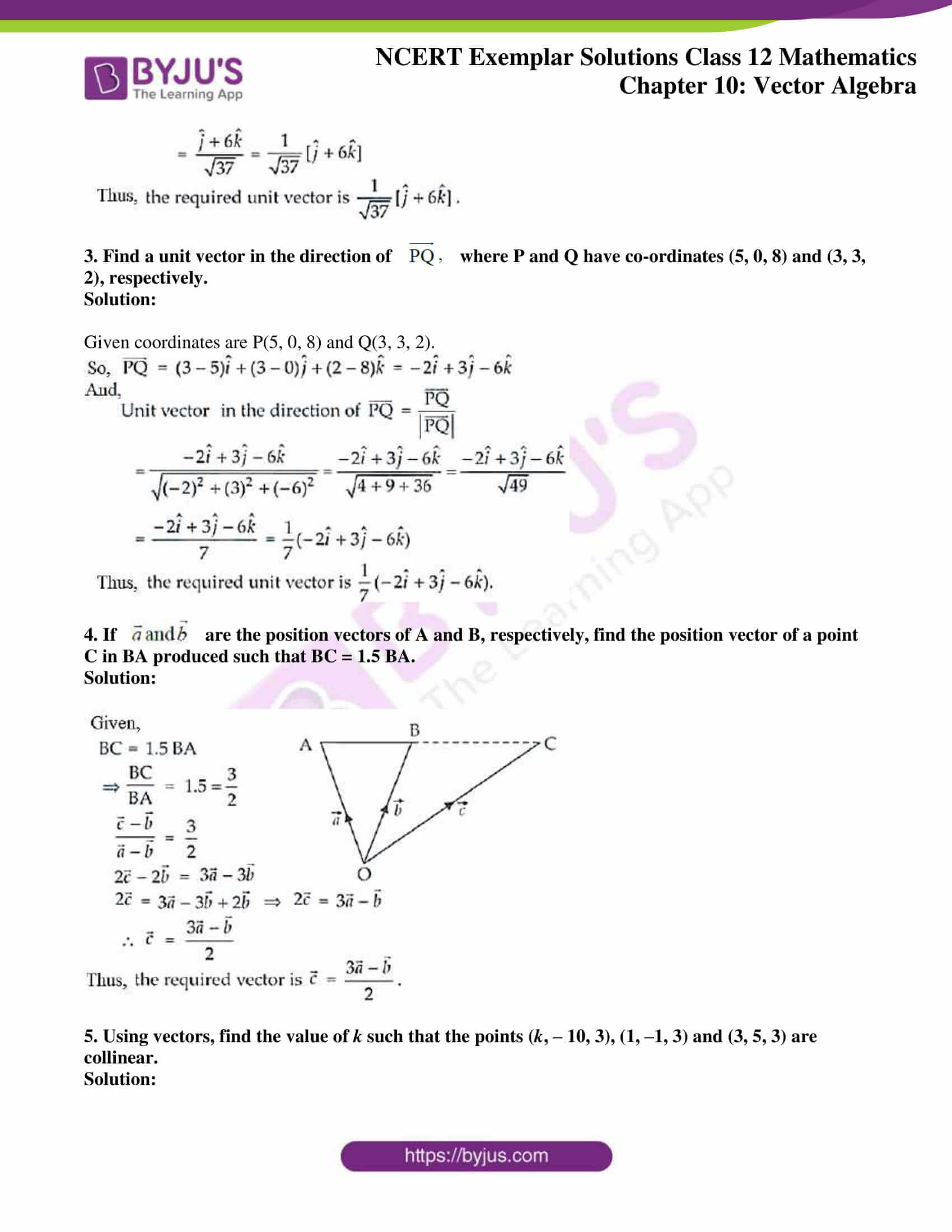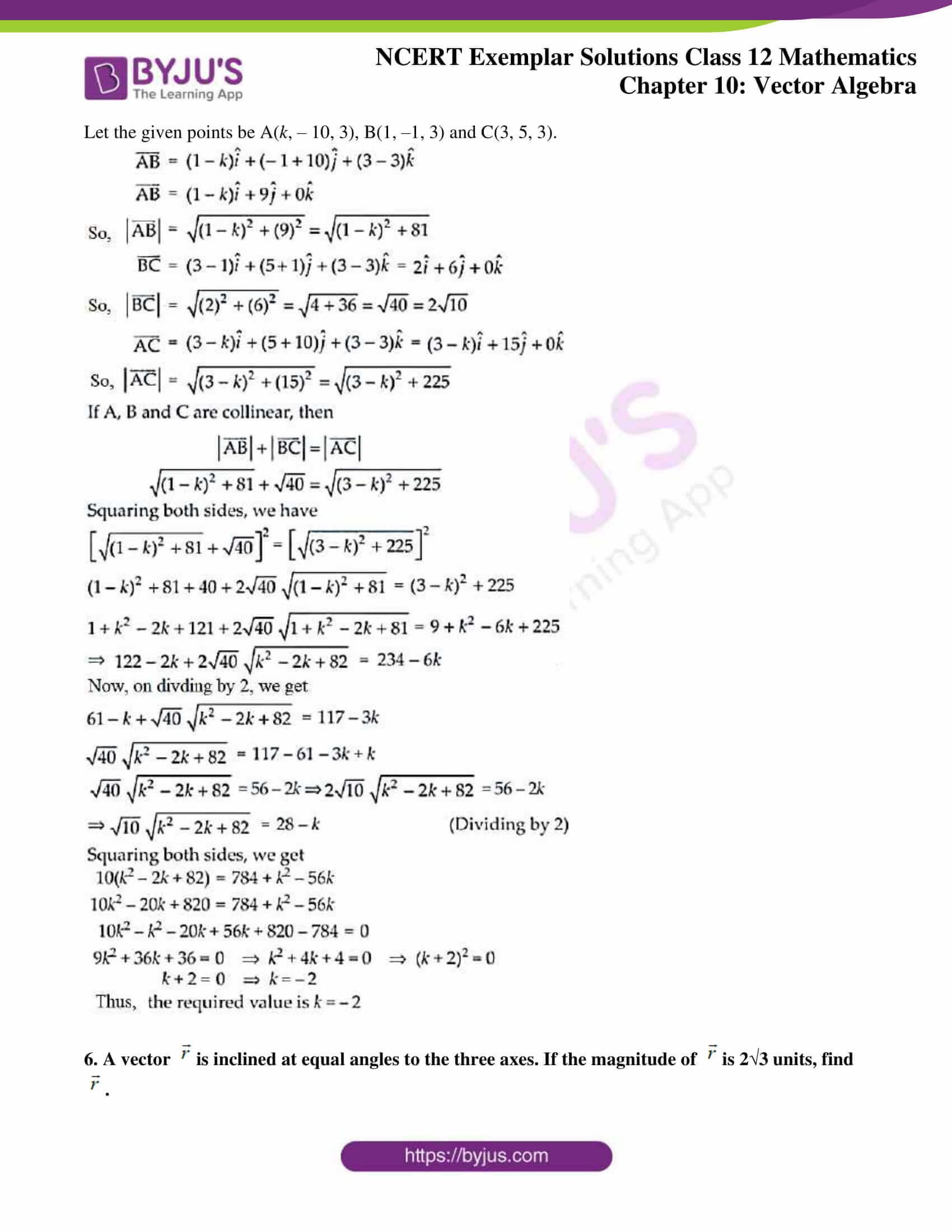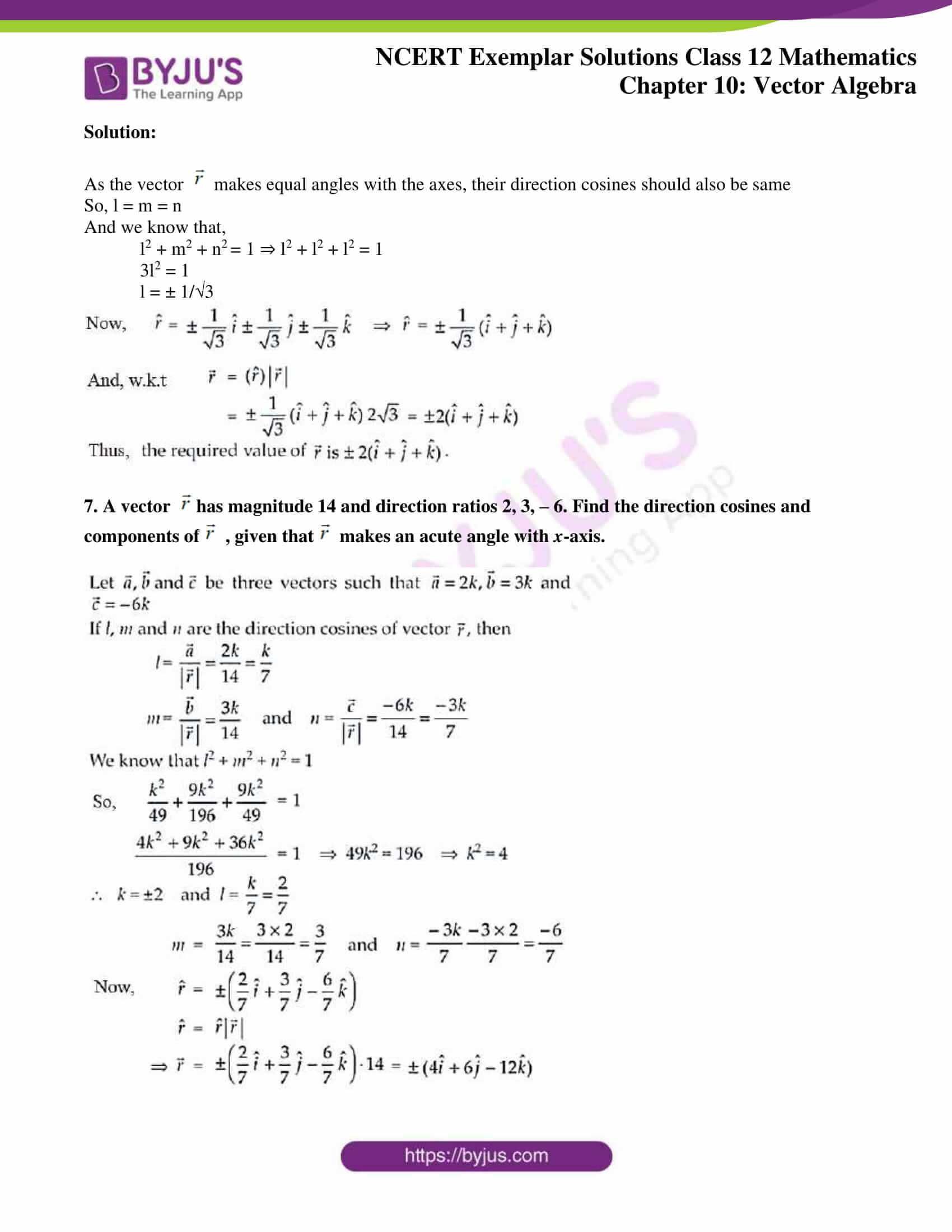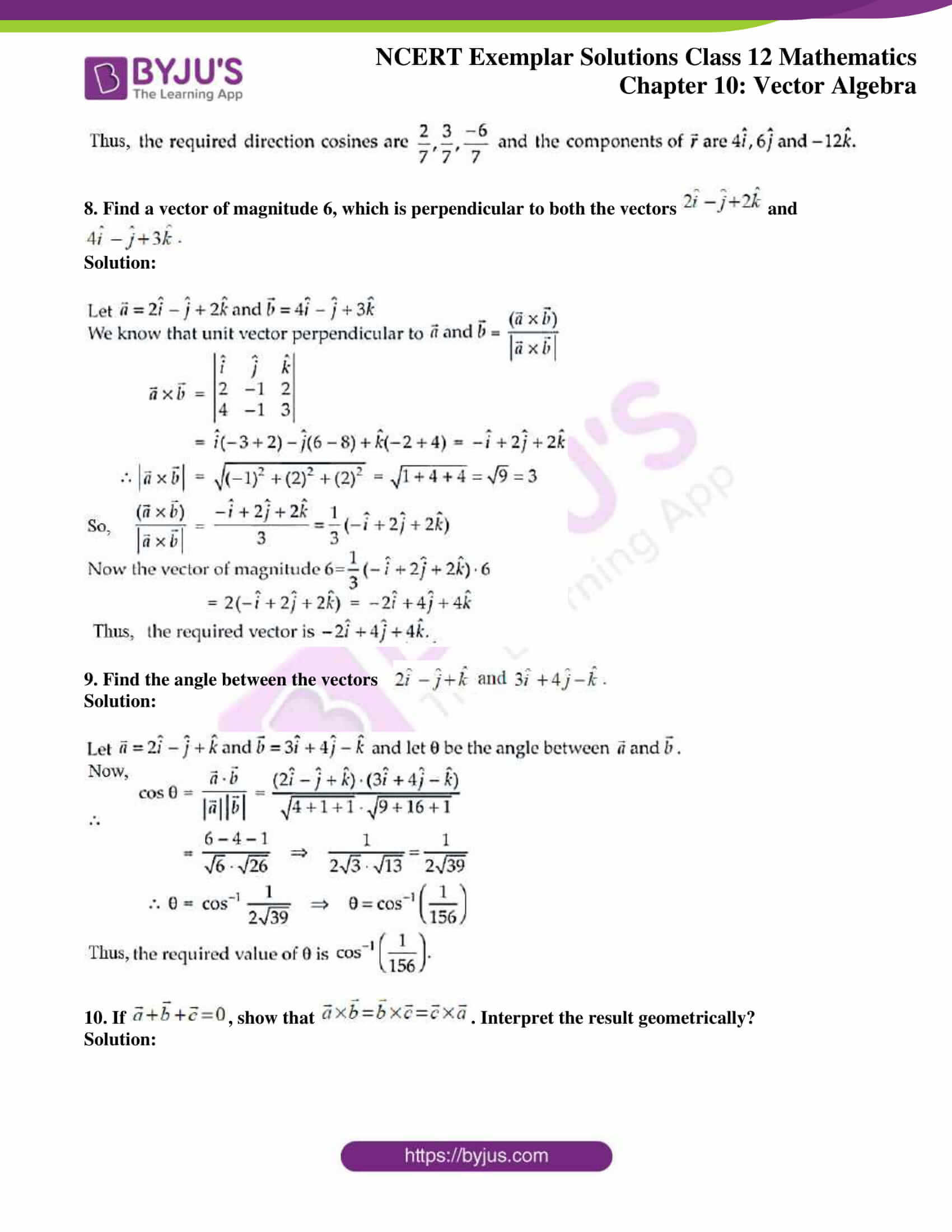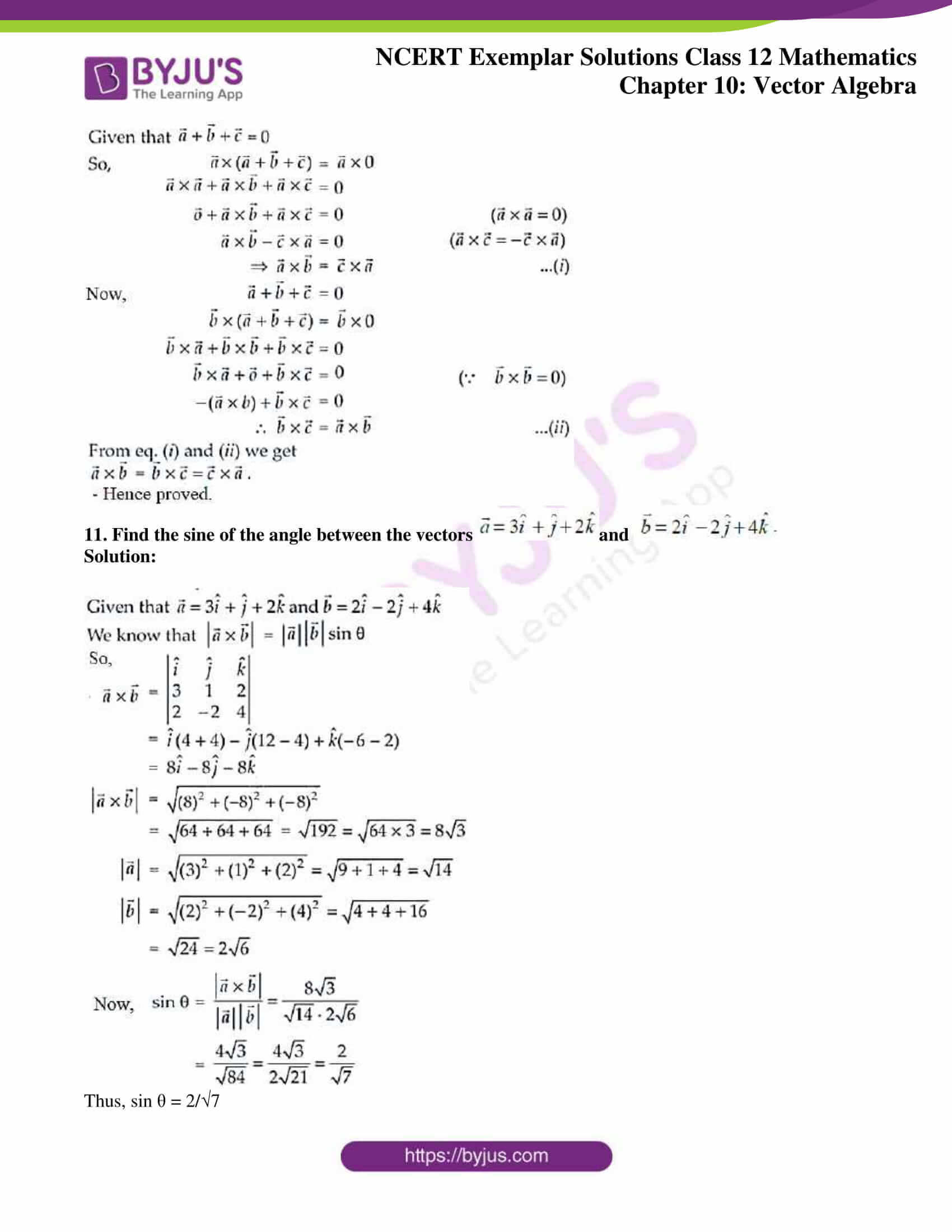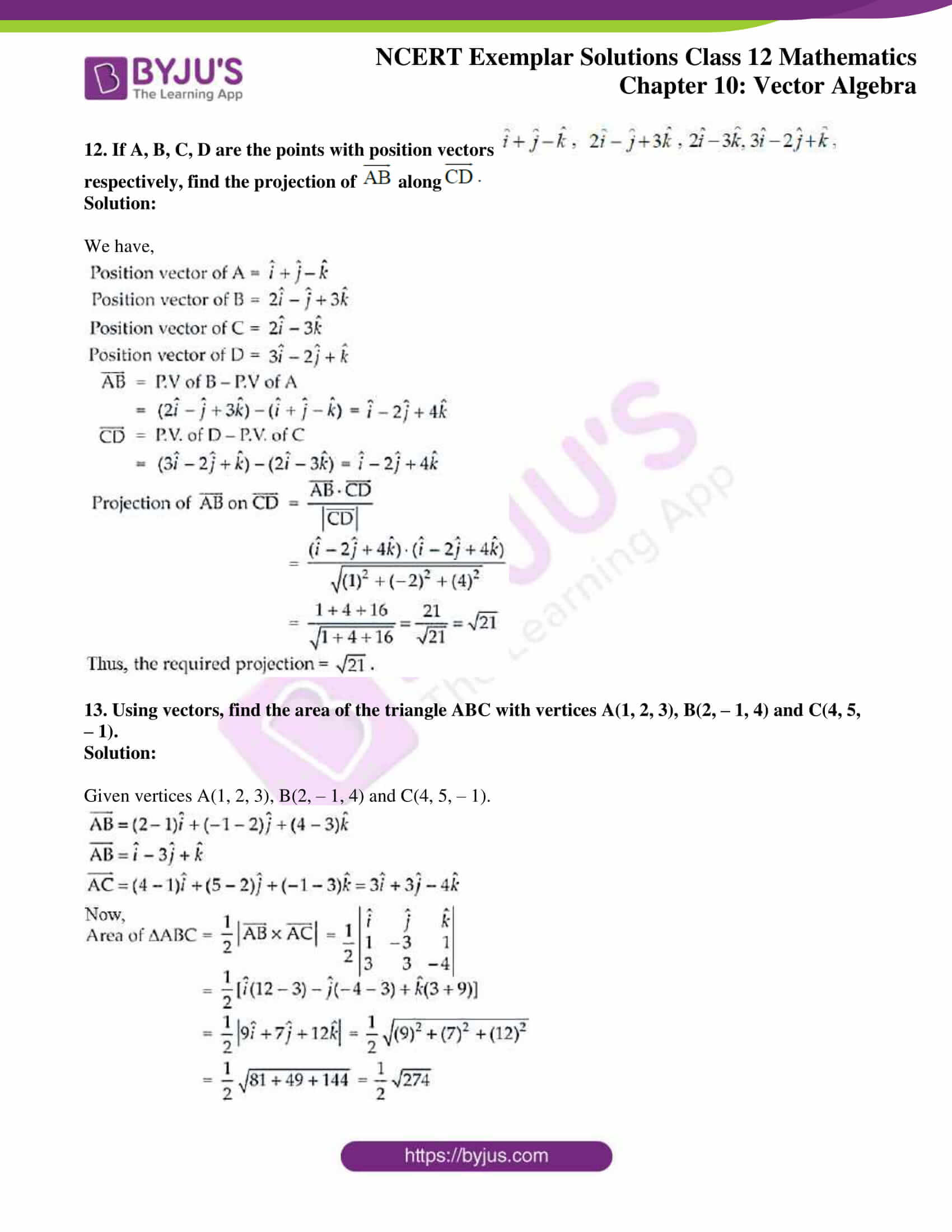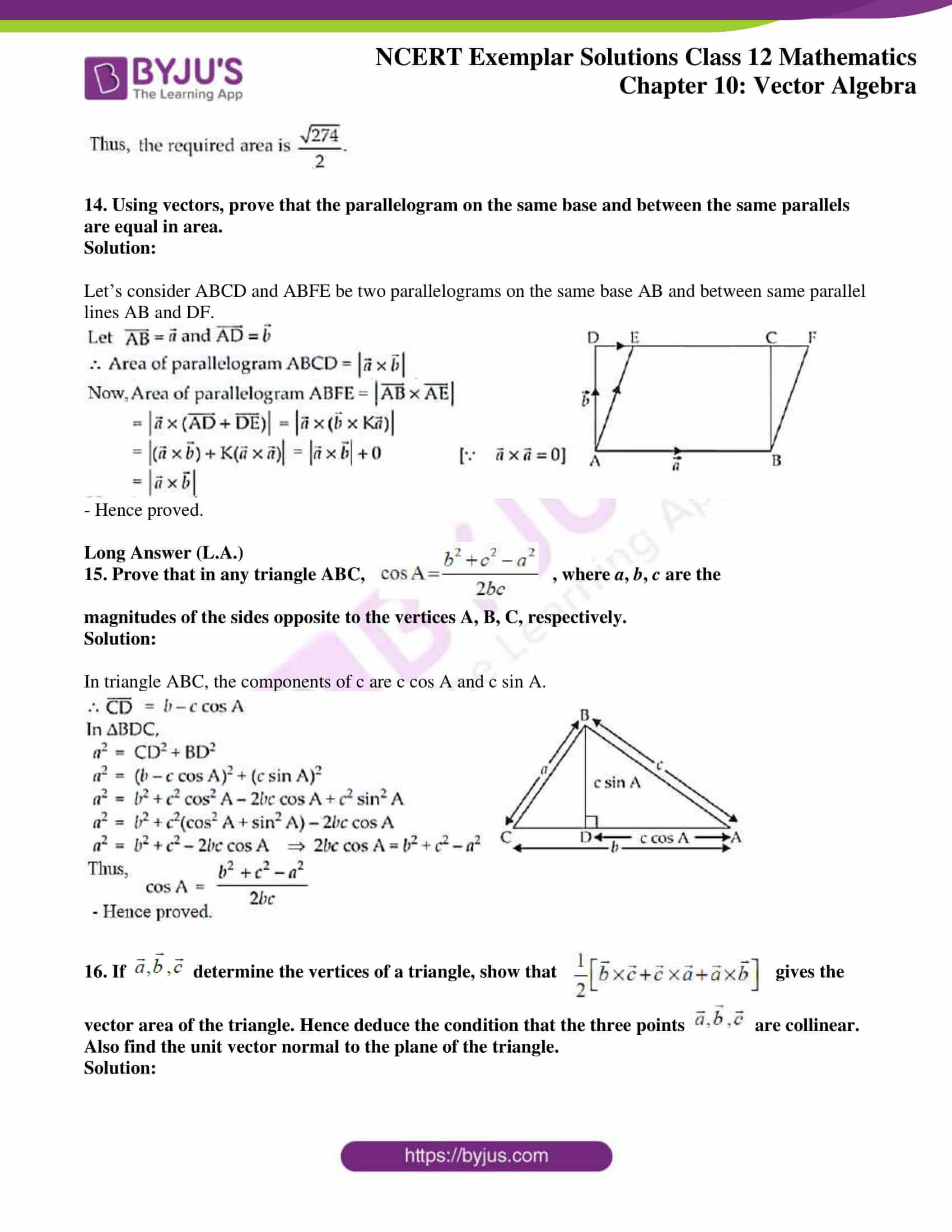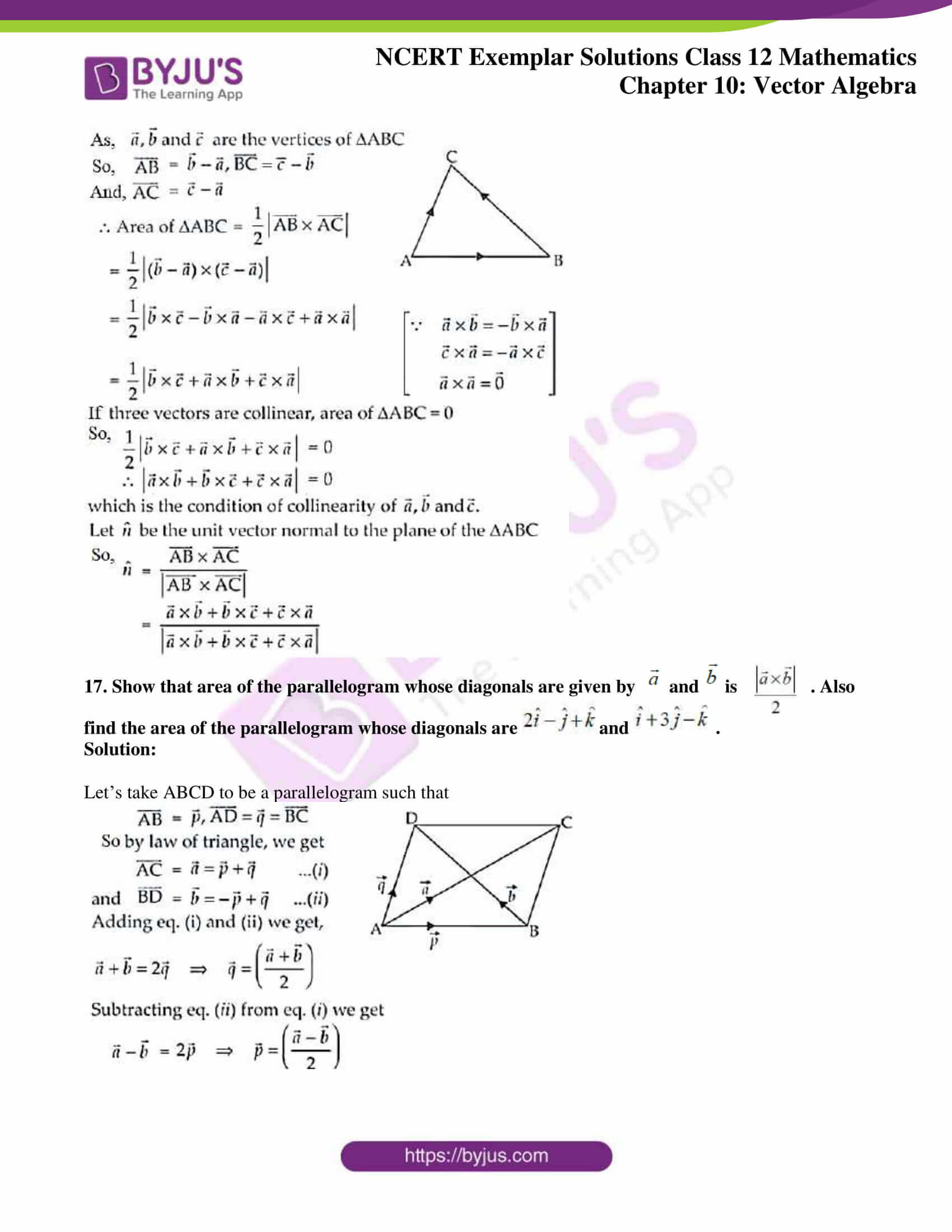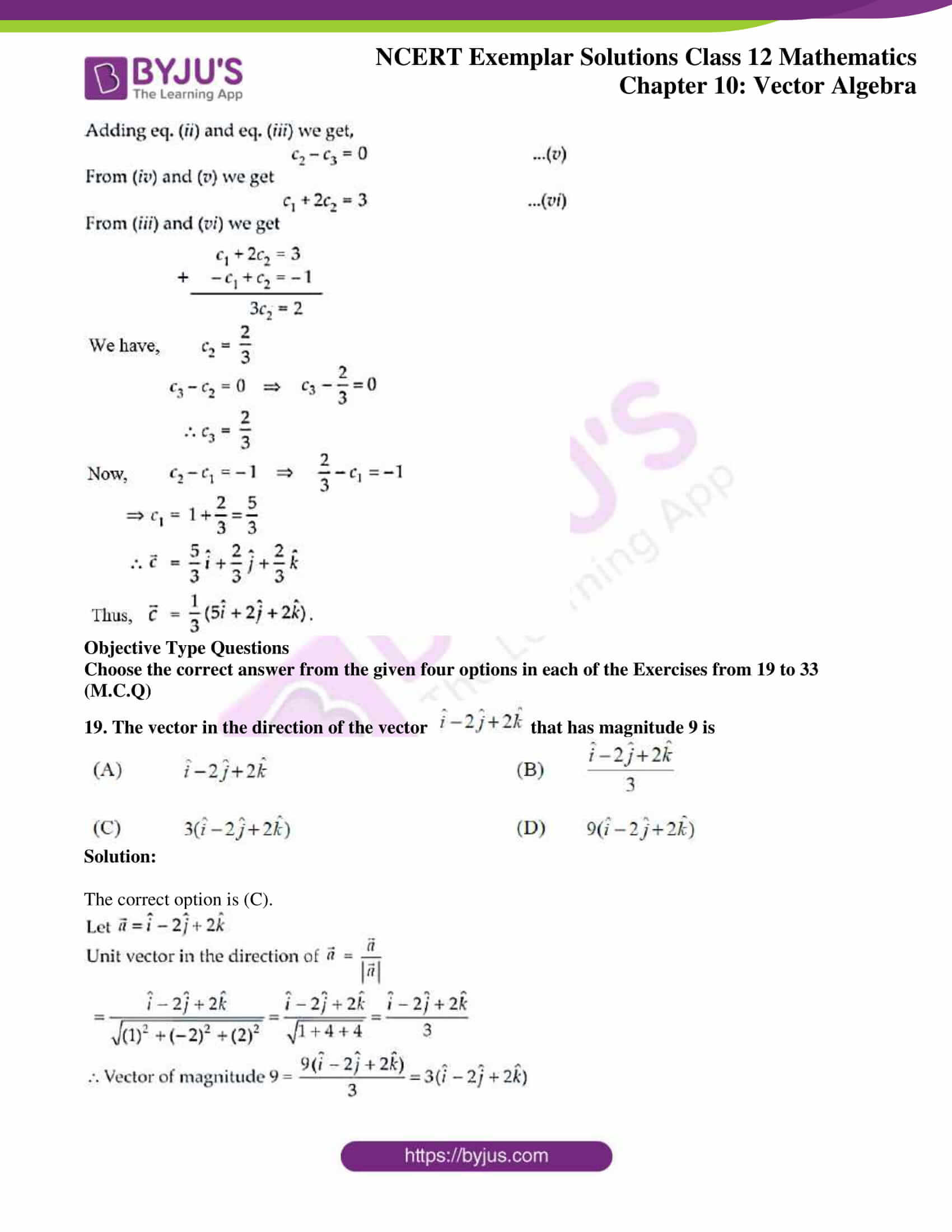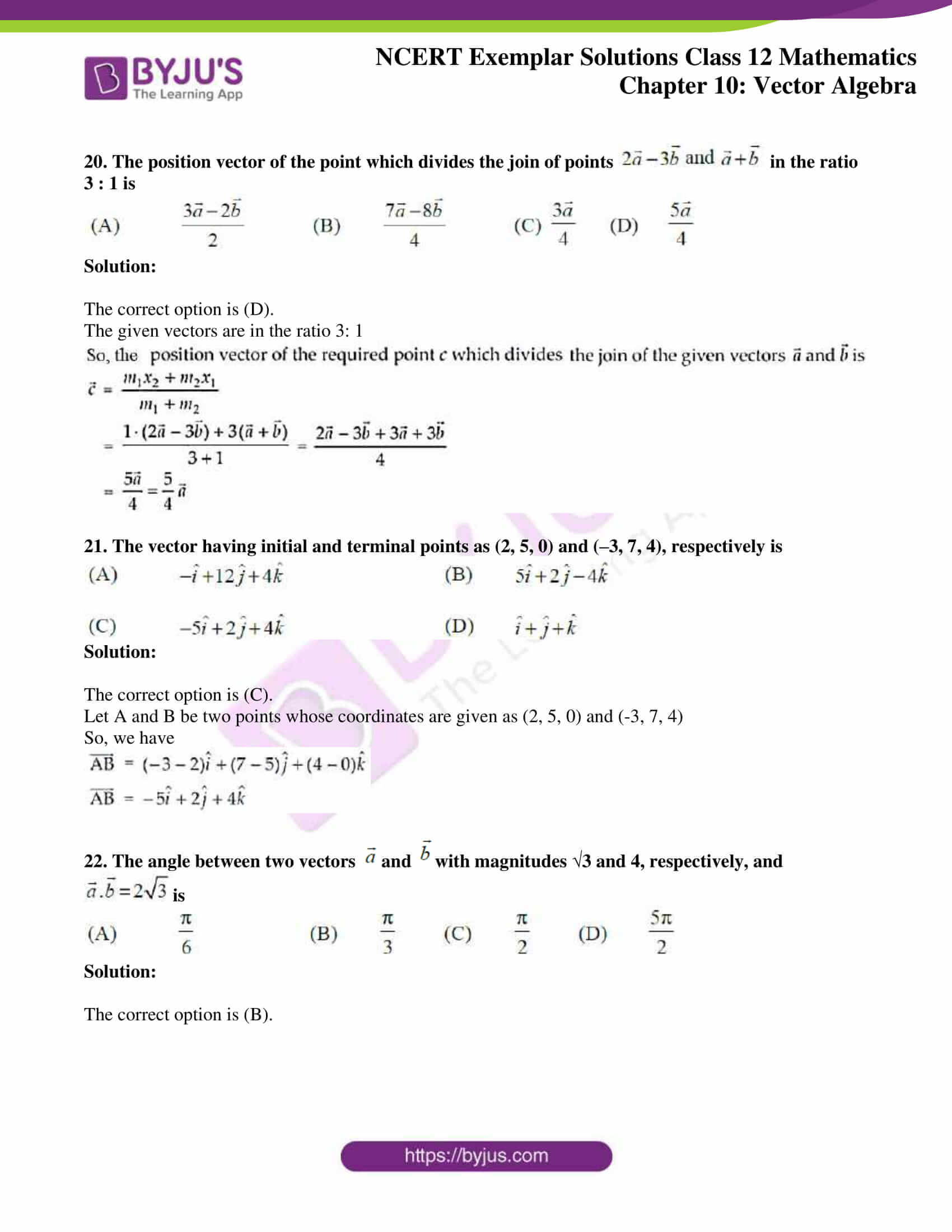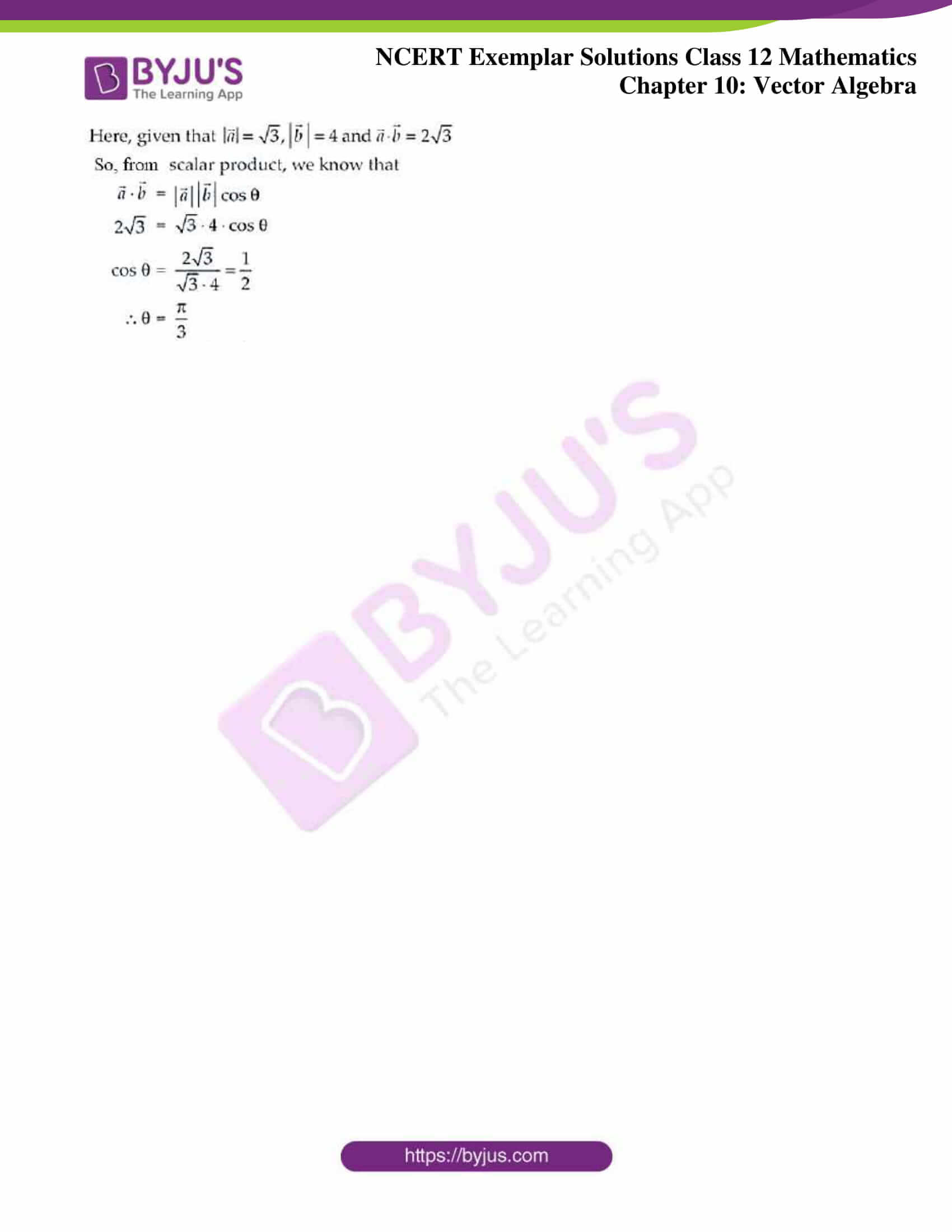### Access Answers to the NCERT Exemplar Class 12 Maths Chapter 10 Vector Algebra

Exercise 10.3 Page No: 215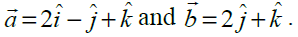1. Find the unit vector in the direction of sum of vectors

Solution:

Given vectors are,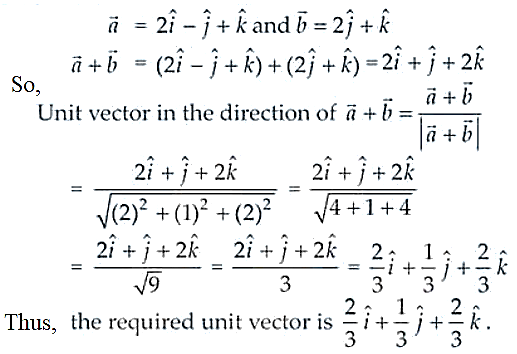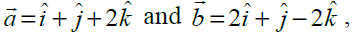2. If find the unit vector in the direction of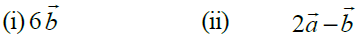Solution: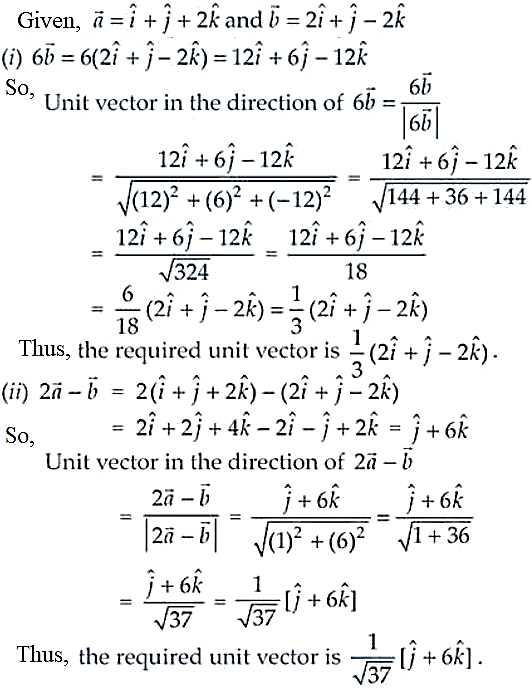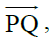3. Find a unit vector in the direction of where P and Q have co-ordinates (5, 0, 8) and (3, 3, 2), respectively.

Solution:

Given coordinates are P(5, 0, 8) and Q(3, 3, 2).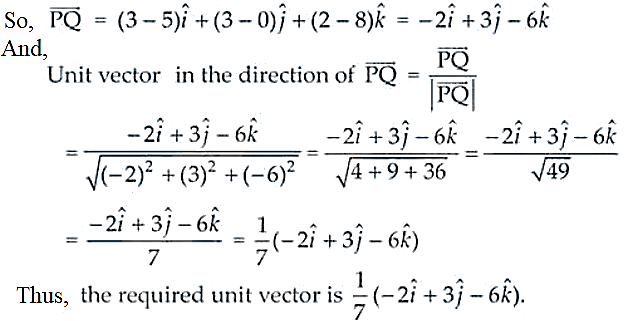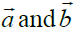4. If are the position vectors of A and B, respectively, find the position vector of a point C in BA produced such that BC = 1.5 BA.

Solution: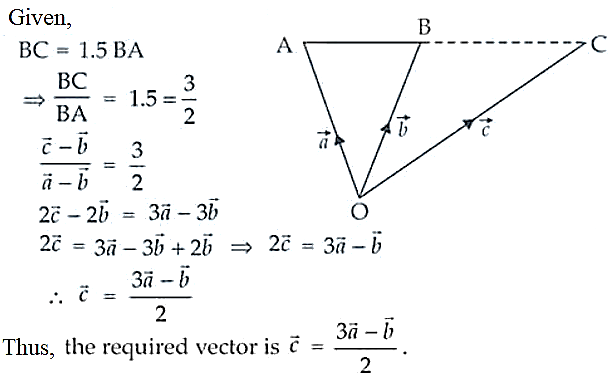5. Using vectors, find the value of k such that the points (k, – 10, 3), (1, –1, 3) and (3, 5, 3) are collinear.

Solution:

Let the given points be A(k, – 10, 3), B(1, –1, 3) and C(3, 5, 3).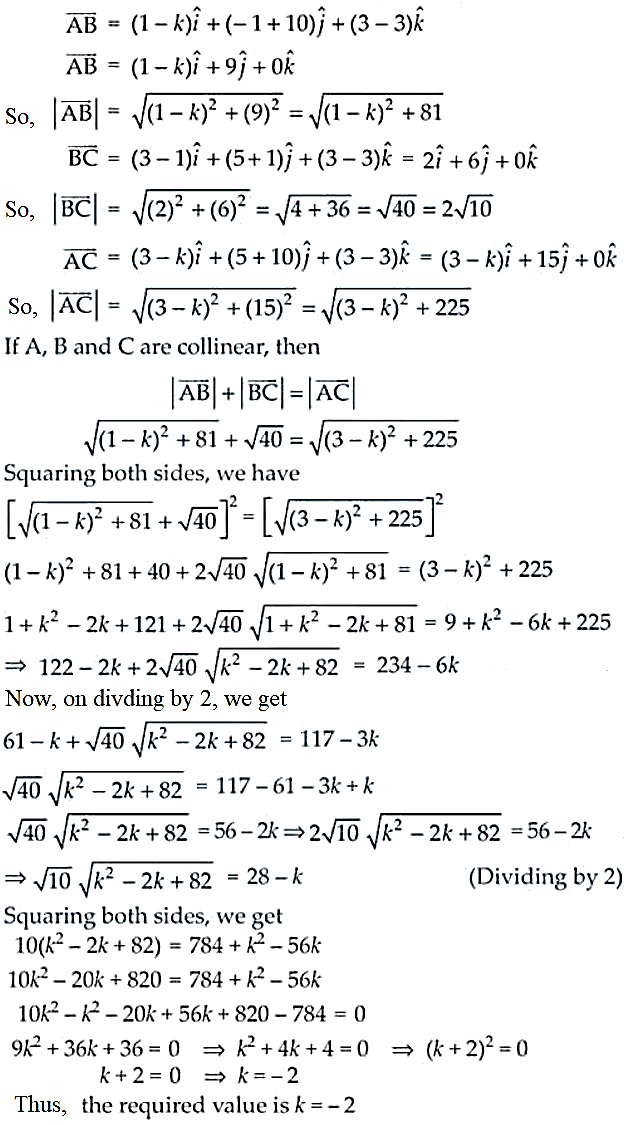6. A vector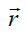is inclined at equal angles to the three axes. If the magnitude ofis 2√3 units, find.

Solution:

As the vectormakes equal angles with the axes, their direction cosines should also be same

So, l = m = n

And we know that,

l2 + m2 + n2 = 1 ⇒ l2 + l2 + l2 = 1

3l2 = 1

l = ± 1/√3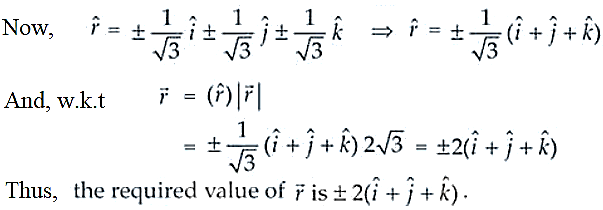7. A vector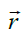has magnitude 14 and direction ratios 2, 3, – 6. Find the direction cosines and components of, given thatmakes an acute angle with x-axis.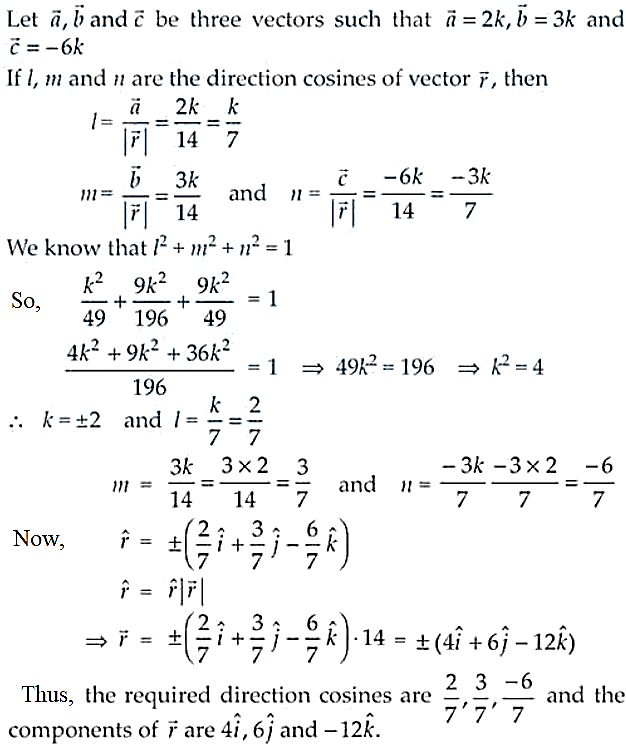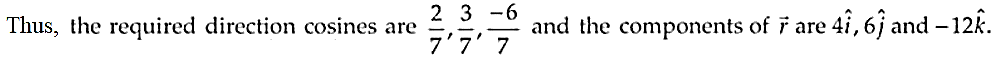8. Find a vector of magnitude 6, which is perpendicular to both the vectors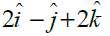and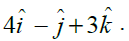Solution: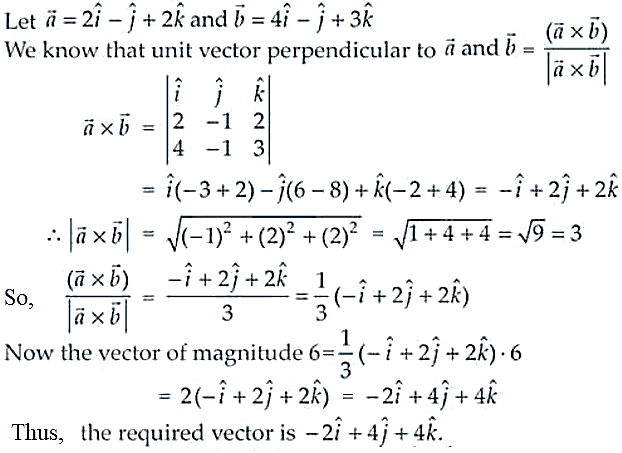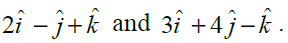9. Find the angle between the vectors

Solution: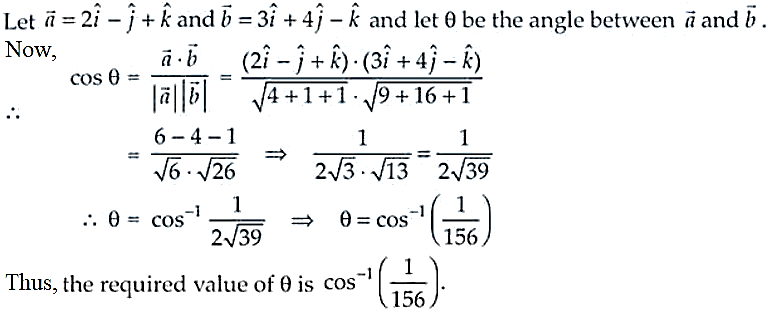10. If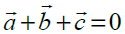, show that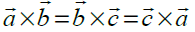. Interpret the result geometrically?

Solution: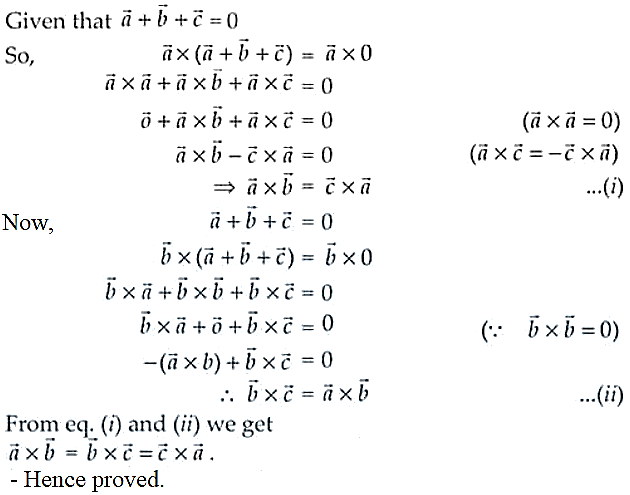11. Find the sine of the angle between the vectors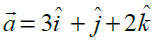and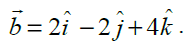Solution: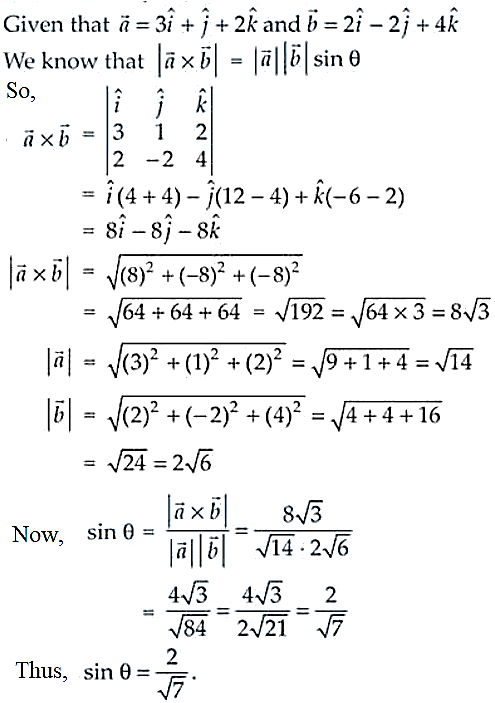Thus, sin θ = 2/√7

12. If A, B, C, D are the points with position vectors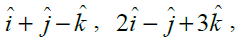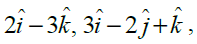respectively, find the projection of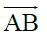along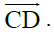Solution:

We have,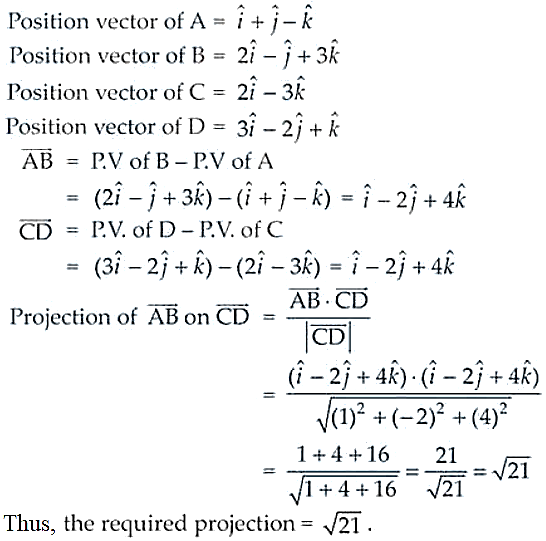13. Using vectors, find the area of the triangle ABC with
vertices A(1, 2, 3), B(2, – 1, 4) and C(4, 5, – 1).

Solution:

Given vertices A(1, 2, 3), B(2, – 1, 4) and C(4, 5, – 1).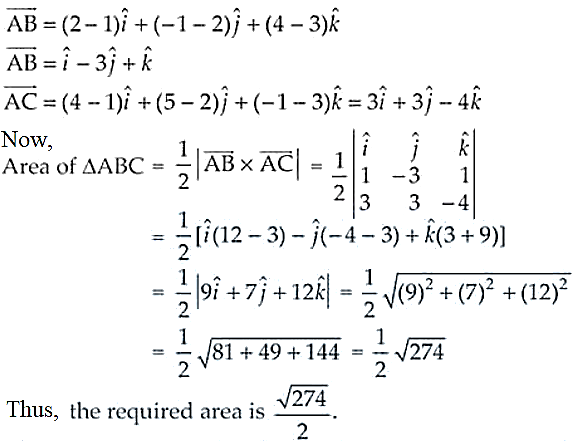14. Using vectors, prove that the parallelogram on the same base and between the same parallels are equal in area.

Solution:

Let’s consider ABCD and ABFE be two parallelograms on the same base AB and between same parallel lines AB and DF.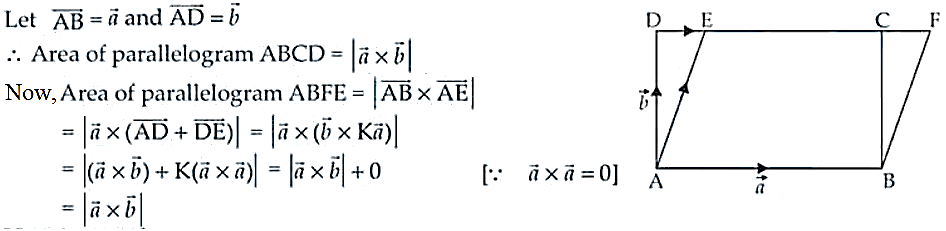– Hence proved.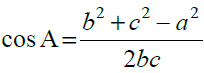Long Answer (L.A.)

15. Prove that in any triangle ABC, , where a, b, c are the

magnitudes of the sides opposite to the vertices A, B, C, respectively.

Solution:

In triangle ABC, the components of c are c cos A and c sin A.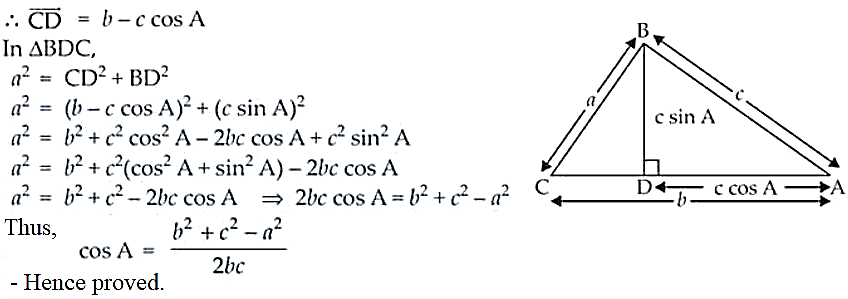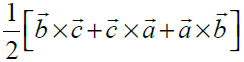16. If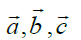determine the vertices of a triangle, show that gives the

vector area of the triangle. Hence deduce the condition that the three points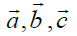are collinear. Also find the unit vector normal to the plane of the triangle.

Solution: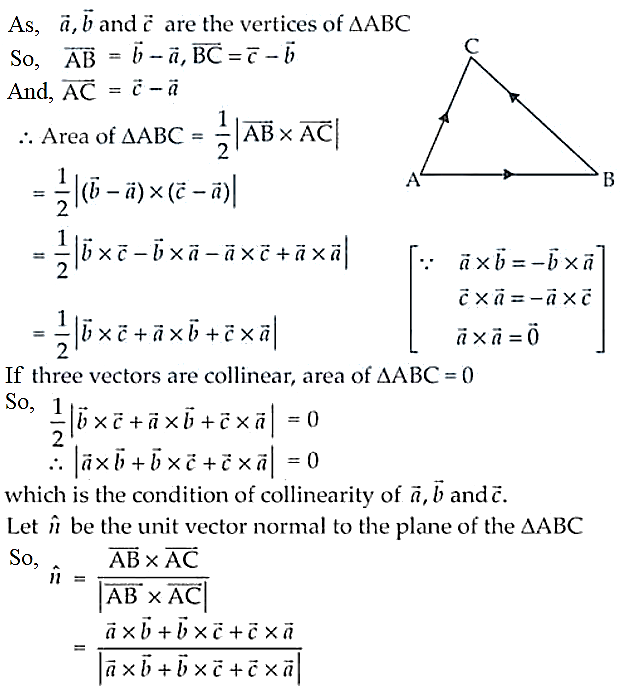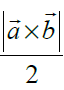17. Show that area of the parallelogram whose diagonals are given by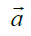and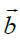is . Also find the area of the parallelogram whose diagonals are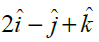and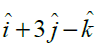.

Solution:

Let’s take ABCD to be a parallelogram such that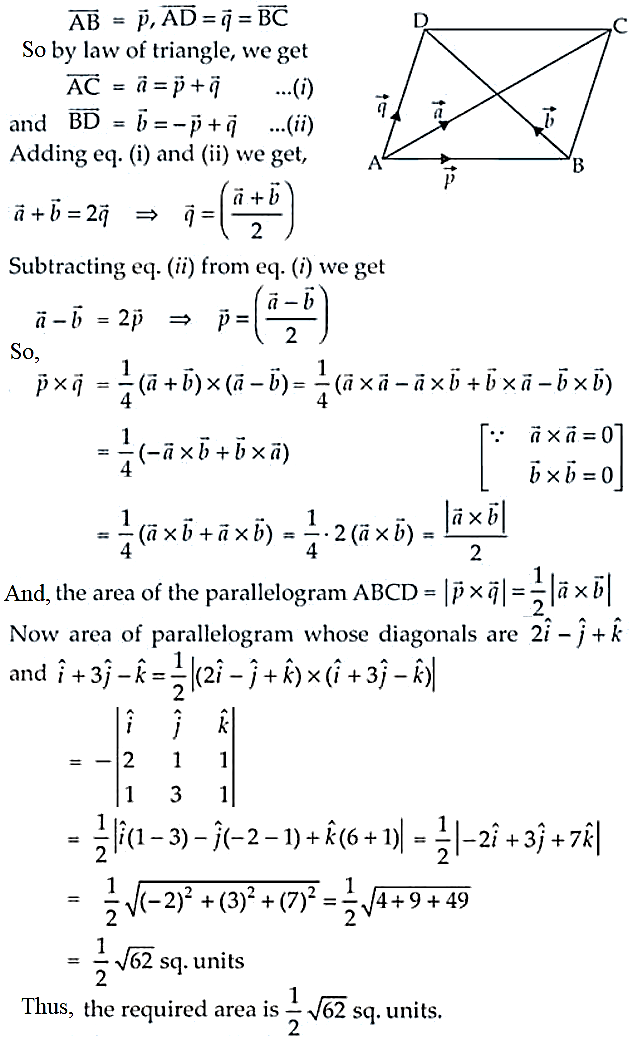18. If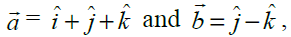find a vector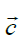such that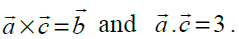Solution: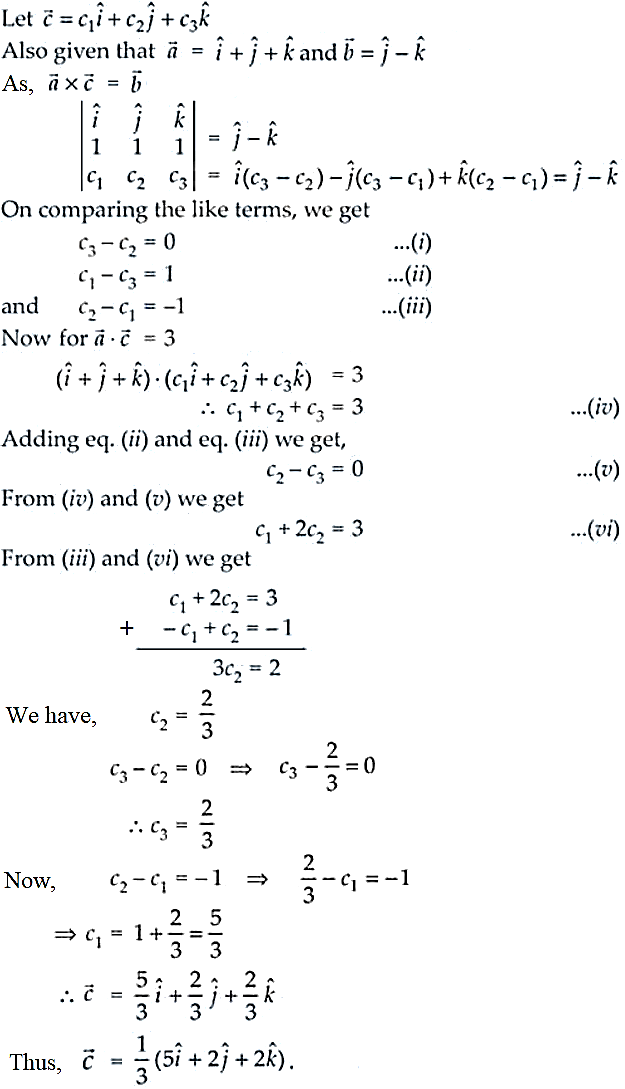Objective Type Questions

Choose the correct answer from the given four options in each of the Exercises from 19 to 33 (M.C.Q)

19. The vector in the direction of the vector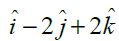that has magnitude 9 is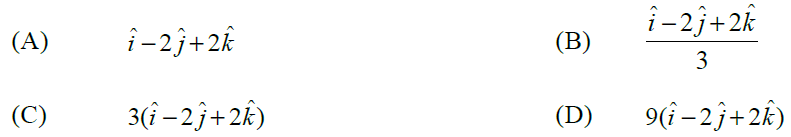Solution:

The correct option is (C).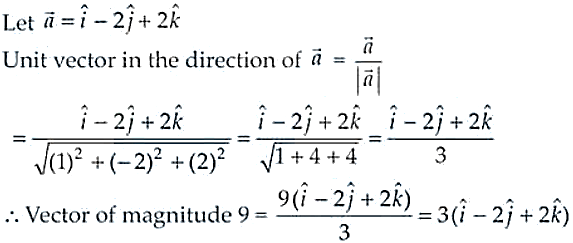20. The position vector of the point which divides the join of points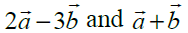in the ratio 3 : 1 is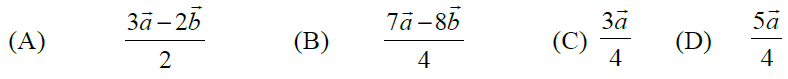Solution:

The correct option is (D).

The given vectors are in the ratio 3: 1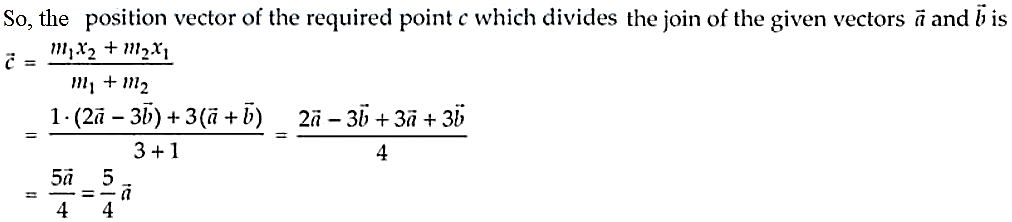21. The vector having initial and terminal points as (2, 5, 0) and (–3, 7, 4), respectively is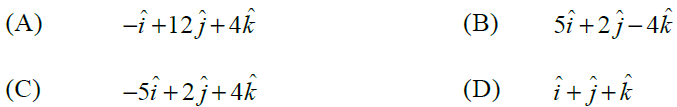Solution:

The correct option is (C).

Let A and B be two points whose coordinates are given as (2, 5, 0) and (-3, 7, 4)

So, we have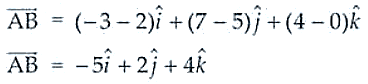22. The angle between two vectors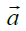and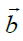with magnitudes √3 and 4, respectively, and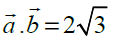is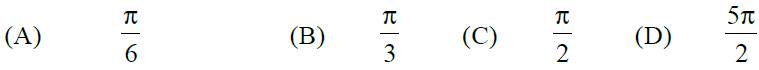Solution:

The correct option is (B).